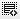## Difference between pd.Series([[10,20,500]]) and pd.Series([10,20,50])0

import pandas as pd

# dict to dataframe
dic = {'User':['u1', 'u2', 'u3', 'u3'],'Age':[10, 20, 30, 40],'Gender':["M","F","M","F"]}
df = pd.DataFrame(dic) # with default index

# Add column 'Weight' using 1D list
df['Weight'] = pd.Series([10,20,50])
print("Updated DF using series:\n", df,"\n")
# In the above case it is converting a 1d list to a 1d Series with [10,20,500] as first three elements, so [10,20,500] is assigned from index 0 to index 2 and index 3 is defined as NaN

# Add column 'Weight' using 2D list
df['Weight'] = pd.Series([[10,20,500]])
print("Updated DF using series:\n", df,"\n")
# But in the above case it is converting a 1d sublist to a 1d Series with [10,20,500] as one of the elements in the list, so [10,20,500] is assigned to index 0 and rest of the indices are defined as NaN

# Try Note:
lis1d = [10, 20, 50]
s1d = pd.Series(lis1d)
print("\n Size of Series:", s1d.shape)
print("1D series from 1D list: \n", s1d)

lis1d_sub = [[10, 20, 50],] # it is equivalent to [ [10, 20, 50] , ]
s2_sub = pd.Series(lis1d_sub)
print("\n Size of Series:", s2_sub.shape)
print("1D series from 1D sublist: \n", s2_sub)

Click on thisicon to add code snippet.